# linearequns-classx-180912070018.pdf

27. Sep 2022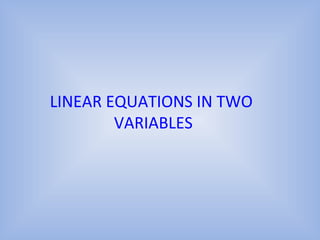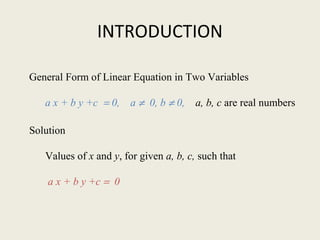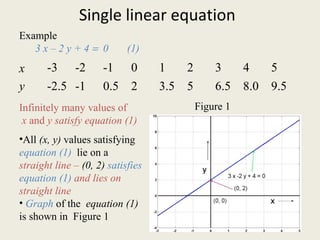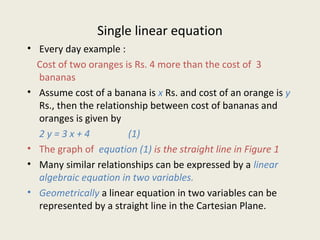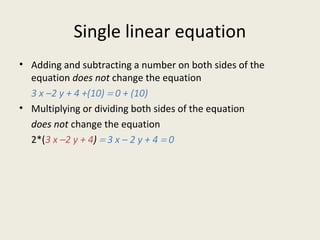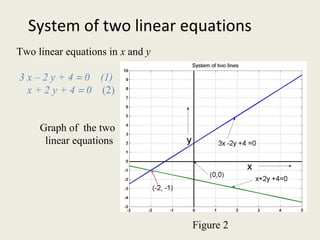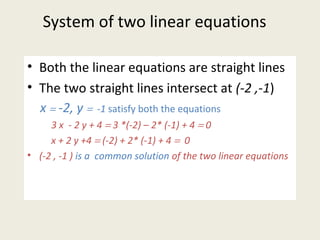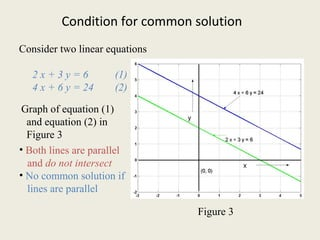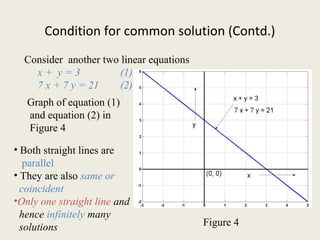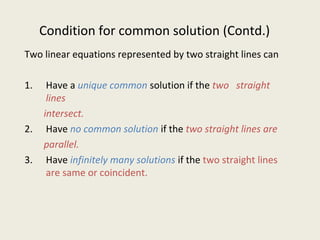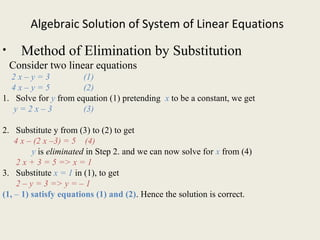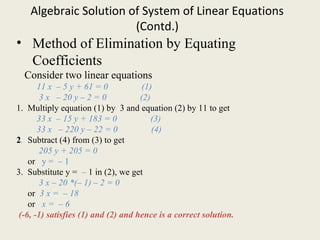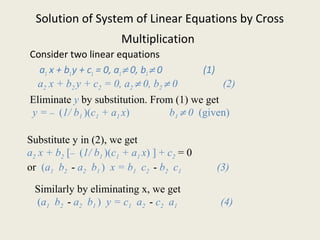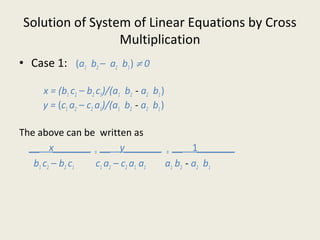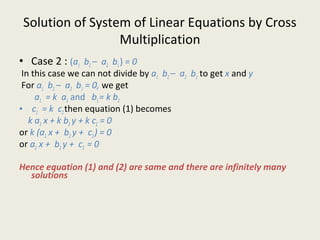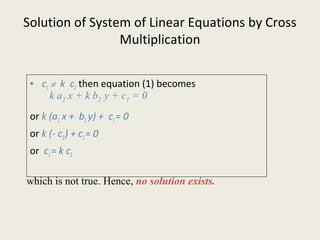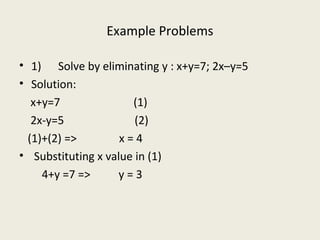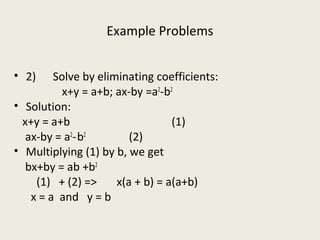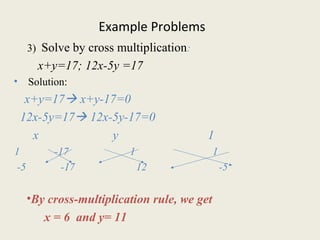1 von 20

### linearequns-classx-180912070018.pdf

• 1. LINEAR EQUATIONS IN TWO VARIABLES
• 2. INTRODUCTION General Form of Linear Equation in Two Variables a x + b y +c = 0, a ≠ 0, b ≠ 0, a, b, c are real numbers Solution Values of x and y, for given a, b, c, such that a x + b y +c = 0
• 3. Single linear equation Example 3 x – 2 y + 4 = 0 (1) Infinitely many values of x and y satisfy equation (1) x -3 -2 -1 0 1 2 3 4 5 y -2.5 -1 0.5 2 3.5 5 6.5 8.0 9.5 •All (x, y) values satisfying equation (1) lie on a straight line – (0, 2) satisfies equation (1) and lies on straight line • Graph of the equation (1) is shown in Figure 1 Figure 1
• 4. Single linear equation • Every day example : Cost of two oranges is Rs. 4 more than the cost of 3 bananas • Assume cost of a banana is x Rs. and cost of an orange is y Rs., then the relationship between cost of bananas and oranges is given by 2 y = 3 x + 4 (1) • The graph of equation (1) is the straight line in Figure 1 • Many similar relationships can be expressed by a linear algebraic equation in two variables. • Geometrically a linear equation in two variables can be represented by a straight line in the Cartesian Plane.
• 5. Single linear equation • Adding and subtracting a number on both sides of the equation does not change the equation 3 x –2 y + 4 +(10) = 0 + (10) • Multiplying or dividing both sides of the equation does not change the equation 2*(3 x –2 y + 4) = 3 x – 2 y + 4 = 0
• 6. System of two linear equations Two linear equations in x and y 3 x – 2 y + 4 = 0 (1) x + 2 y + 4 = 0 (2) Graph of the two linear equations Figure 2
• 7. System of two linear equations • Both the linear equations are straight lines • The two straight lines intersect at (-2 ,-1) x = -2, y = -1 satisfy both the equations 3 x - 2 y + 4 = 3 *(-2) – 2* (-1) + 4 = 0 x + 2 y +4 = (-2) + 2* (-1) + 4 = 0 • (-2 , -1 ) is a common solution of the two linear equations
• 8. Condition for common solution Consider two linear equations 2 x + 3 y = 6 (1) 4 x + 6 y = 24 (2) • Both lines are parallel and do not intersect • No common solution if lines are parallel Figure 3 Graph of equation (1) and equation (2) in Figure 3
• 9. Condition for common solution (Contd.) Consider another two linear equations x + y = 3 (1) 7 x + 7 y = 21 (2) Figure 4 Graph of equation (1) and equation (2) in Figure 4 • Both straight lines are parallel • They are also same or coincident •Only one straight line and hence infinitely many solutions
• 10. Condition for common solution (Contd.) Two linear equations represented by two straight lines can 1. Have a unique common solution if the two straight lines intersect. 2. Have no common solution if the two straight lines are parallel. 3. Have infinitely many solutions if the two straight lines are same or coincident.
• 11. Algebraic Solution of System of Linear Equations • Method of Elimination by Substitution Consider two linear equations 2 x – y = 3 (1) 4 x – y = 5 (2) 1. Solve for y from equation (1) pretending x to be a constant, we get y = 2 x – 3 (3) 2. Substitute y from (3) to (2) to get 4 x – (2 x –3) = 5 (4) y is eliminated in Step 2. and we can now solve for x from (4) 2 x + 3 = 5 => x = 1 3. Substitute x = 1 in (1), to get 2 – y = 3 => y = – 1 (1, – 1) satisfy equations (1) and (2). Hence the solution is correct.
• 12. Algebraic Solution of System of Linear Equations (Contd.) • Method of Elimination by Equating Coefficients Consider two linear equations 11 x – 5 y + 61 = 0 (1) 3 x – 20 y – 2 = 0 (2) 1. Multiply equation (1) by 3 and equation (2) by 11 to get 33 x – 15 y + 183 = 0 (3) 33 x – 220 y – 22 = 0 (4) 2. Subtract (4) from (3) to get 205 y + 205 = 0 or y = – 1 3. Substitute y = – 1 in (2), we get 3 x – 20 *(– 1) – 2 = 0 or 3 x = – 18 or x = – 6 (-6, -1) satisfies (1) and (2) and hence is a correct solution.
• 13. Solution of System of Linear Equations by Cross Multiplication Consider two linear equations a1 x + b1y + c1 = 0, a1≠ 0, b1≠ 0 (1) a2 x + b2 y + c2 = 0, a2 ≠ 0, b2 ≠ 0 (2) Eliminate y by substitution. From (1) we get y = – (1/ b1 )(c1 + a1 x) b1 ≠ 0 (given) Substitute y in (2), we get a2 x + b2 [– (1/ b1 )(c1 + a1 x) ] + c2 = 0 or (a1 b2 - a2 b1 ) x = b1 c2 - b2 c1 (3) Similarly by eliminating x, we get (a1 b2 - a2 b1 ) y = c1 a2 - c2 a1 (4)
• 14. Solution of System of Linear Equations by Cross Multiplication • Case 1: (a1 b2 – a2 b1) ≠ 0 x = (b1 c2 – b2 c1)/(a1 b2 - a2 b1) y = (c1 a2 – c2 a1)/(a1 b2 - a2 b1) The above can be written as __ x_______ = __ y_______ = __ 1_______ b1 c2 – b2 c1 c1 a2 – c2 a1 a1 a1 b2 - a2 b1
• 15. Solution of System of Linear Equations by Cross Multiplication • Case 2 : (a1 b2 – a2 b1) = 0 In this case we can not divide by a1 b2 – a2 b1 to get x and y For a1 b2 – a2 b1 = 0, we get a1 = k a2 and b1= k b2 • c1 = k c2then equation (1) becomes k a2 x + k b2 y + k c2 = 0 or k (a2 x + b2 y + c2) = 0 or a2 x + b2 y + c2 = 0 Hence equation (1) and (2) are same and there are infinitely many solutions
• 16. Solution of System of Linear Equations by Cross Multiplication • c1 ≠ k c2 then equation (1) becomes or k (a2 x + b2 y) + c1= 0 or k (- c2) + c1= 0 or c1= k c2 k a2 x + k b2 y + c1 = 0 which is not true. Hence, no solution exists.
• 17. Example Problems • 1) Solve by eliminating y : x+y=7; 2x–y=5 • Solution: x+y=7 (1) 2x-y=5 (2) (1)+(2) => x = 4 • Substituting x value in (1) 4+y =7 => y = 3
• 18. Example Problems • 2) Solve by eliminating coefficients: x+y = a+b; ax-by =a2 -b2 • Solution: x+y = a+b (1) ax-by = a2 -b2 (2) • Multiplying (1) by b, we get bx+by = ab +b2 (1) + (2) => x(a + b) = a(a+b) x = a and y = b
• 19. Example Problems 3) Solve by cross multiplication: x+y=17; 12x-5y =17 • Solution: x+y=17 x+y-17=0 12x-5y=17 12x-5y-17=0 x y 1 1 -17 1 1 -5 -17 12 -5 •By cross-multiplication rule, we get  x = 6 and y= 11
• 20. THANK YOU